# KSEEB Solutions for Class 8 Maths Chapter 7 Rational Numbers Ex 7.3

In this chapter, we provide KSEEB SSLC Class 8 Maths Chapter 7 Rational Numbers Ex 7.3 for English medium students, Which will very helpful for every student in their exams. Students can download the latest KSEEB SSLC Class 8 Maths Chapter 7 Rational Numbers Ex 7.3 pdf, free KSEEB SSLC Class 8 Maths Chapter 7 Rational Numbers Ex 7.3 pdf download. Now you will get step by step solution to each question.

## Karnataka Board Class 8 Maths Chapter 7 Rational Numbers Ex 7.3

1. Name the property indicated in the following:

Question (i)
315+115 = 430

Question (ii)
34×95=2720
Closure property of multiplication.

Question (iii)
5 + 0 = 0 + 5 = 5

Question (iv)
89×1=89
1 is the multiplicative identity

Question (v)
817+−817=0
−817

Question (vi)
2223×2322=1
2322 is the multiplicative identity

2. Check the commutative property of addition for the following pairs.

Question (i)
102201,34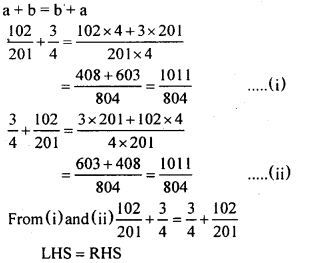Question (ii)
−813,2327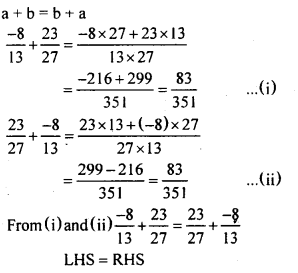Question (iii)
−79,−1819
a + b = b + a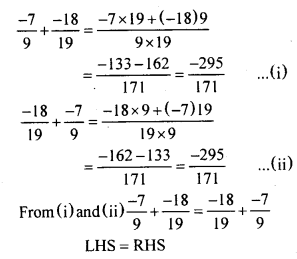3. Check the commutative property of multiplication for the following pairs

Question (i)
2245,34Question (ii)
−713,2527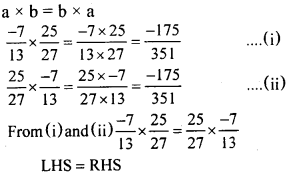Question (iii)
−89,−1719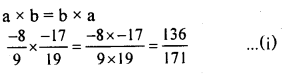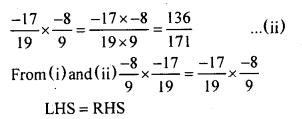4. Check the distributive property for the following triples of rational numbers.

Question (i)
18,19,110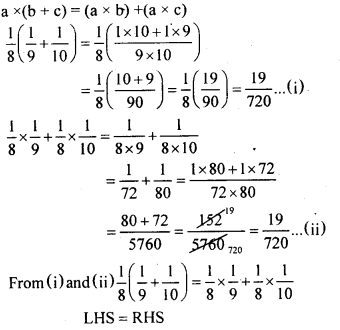Question (ii)
−49,65,1110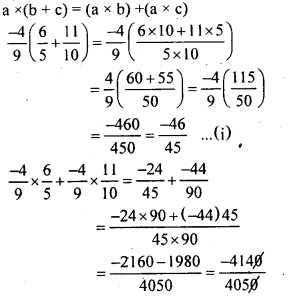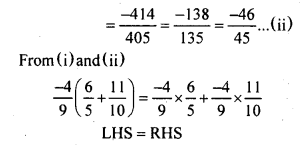Question (iii)
38,0,137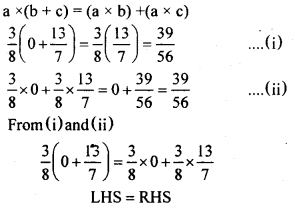5. Find the additive inverse of each of the following numbers.

Question (1)
85
−85

Question (2)
610
−610

Question (3)
−38
38

Question (4)
−163
163

Question (5)
−41
41

6. Find the multiplicative inverse of each of the following numbers.

Question (1)
2
12

Question (2)
611
116

Question (3)
−815
−158

Question (4)
1918
1819

Question (5)
11000
10001

All Chapter KSEEB Solutions For Class 8 maths

—————————————————————————–

All Subject KSEEB Solutions For Class 9

*************************************************

I think you got complete solutions for this chapter. If You have any queries regarding this chapter, please comment on the below section our subject teacher will answer you. We tried our best to give complete solutions so you got good marks in your exam.

If these solutions have helped you, you can also share kseebsolutionsfor.com to your friends.

Best of Luck!!